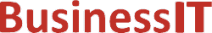O nás     Inzerce     KontaktSpolehlivé informace o IT již od roku 2011[Linux manuál]

# atan2, atan2f, atan2l: obloukové tečné funkce

Originální popis anglicky: atan2, atan2f, atan2l - arc tangent functions

Návod, kniha: POSIX Programmer's Manual

## STRUČNĚ

#include <math.h>

double atan2(double y, double x );

float atan2f(float y, float x);

long double atan2l(long double y, long double x);

## POPIS / INSTRUKCE

These functions shall compute the principal value of the arc tangent of y/ x, using the signs of both arguments to determine the quadrant of the return value.
An application wishing to check for error situations should set errno to zero and call feclearexcept(FE_ALL_EXCEPT) before calling these functions. On return, if errno is non-zero or fetestexcept(FE_INVALID | FE_DIVBYZERO | FE_OVERFLOW | FE_UNDERFLOW) is non-zero, an error has occurred.

## NÁVRATOVÁ HODNOTA

Upon successful completion, these functions shall return the arc tangent of y/ x in the range [-pi,pi] radians.
If y is ±0 and x is < 0, ±pi shall be returned.
If y is ±0 and x is > 0, ±0 shall be returned.
If y is < 0 and x is ±0, -pi/2 shall be returned.
If y is > 0 and x is ±0, pi/2 shall be returned.
If x is 0, a pole error shall not occur.
If either x or y is NaN, a NaN shall be returned.
If the result underflows, a range error may occur and y/ x should be returned.
If y is ±0 and x is -0, ±pi shall be returned.
If y is ±0 and x is +0, ±0 shall be returned.
For finite values of ± y > 0, if x is -Inf, ±pi shall be returned.
For finite values of ± y > 0, if x is +Inf, ±0 shall be returned.
For finite values of x, if y is ±Inf, ±pi/2 shall be returned.
If y is ±Inf and x is -Inf, ±3pi/4 shall be returned.
If y is ±Inf and x is +Inf, ±pi/4 shall be returned.
If both arguments are 0, a domain error shall not occur.

## CHYBY / ERRORY

These functions may fail if:
Range Error
The result underflows.
If the integer expression (math_errhandling & MATH_ERRNO) is non-zero, then errno shall be set to [ERANGE]. If the integer expression (math_errhandling & MATH_ERREXCEPT) is non-zero, then the underflow floating-point exception shall be raised.

The following sections are informative.

None.

## APPLICATION USAGE

On error, the expressions (math_errhandling & MATH_ERRNO) and (math_errhandling & MATH_ERREXCEPT) are independent of each other, but at least one of them must be non-zero.

None.

None.

## SOUVISEJÍCÍ

atan() , feclearexcept() , fetestexcept() , isnan() , tan() , the Base Definitions volume of IEEE Std 1003.1-2001, Section 4.18, Treatment of Error Conditions for Mathematical Functions, <math.h> Portions of this text are reprinted and reproduced in electronic form from IEEE Std 1003.1, 2003 Edition, Standard for Information Technology -- Portable Operating System Interface (POSIX), The Open Group Base Specifications Issue 6, Copyright (C) 2001-2003 by the Institute of Electrical and Electronics Engineers, Inc and The Open Group. In the event of any discrepancy between this version and the original IEEE and The Open Group Standard, the original IEEE and The Open Group Standard is the referee document. The original Standard can be obtained online at http://www.opengroup.org/unix/online.html .
 2003 IEEE/The Open Group
©2011-2021 BusinessIT.cz, ISSN 1805-0522 | Názvy použité v textech mohou být ochrannými známkami příslušných vlastníků.
Provozovatel: Bispiral, s.r.o., kontakt: BusinessIT(at)Bispiral.com | Inzerce: Best Online Media, s.r.o., zuzana@online-media.cz
O vydavateli | Pravidla webu BusinessIT.cz a ochrana soukromí | Používáme účetní program Money S3 | pg(9174)